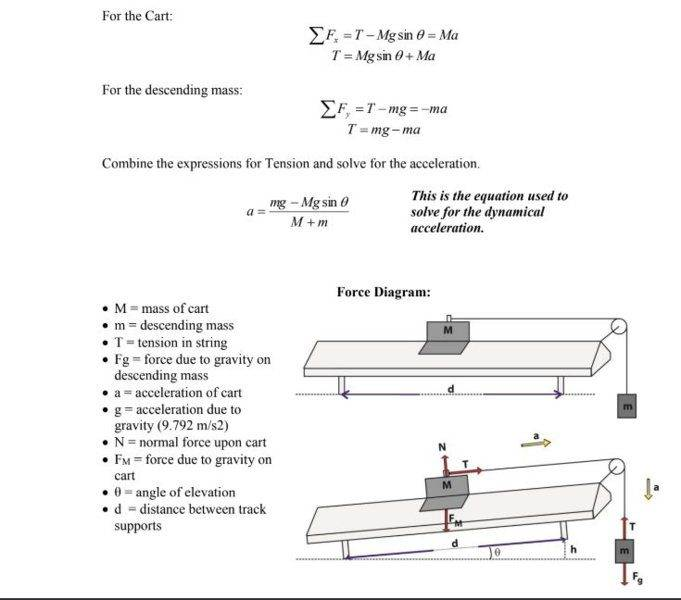# Combine the expressions for tension and solve for acceleration

1. Derive an equation for the acceleration of both the cart and suspended mass in
terms of both masses (Mc and Ms ), the angle of the incline (θ), and the acceleration due to gravity (g).

## Homework Equations

Fx=
T - Mgsin θ = Ma

T = Mgsin θ + Ma
Fy
T = mg - ma

Combine the expressions for Tension and solve for the acceleration.

A= (mg - Mg sin θ / M + m )

https://imgur.com/a/Okk79t9 (I know in the picture it's already derived in the correct form I just want to know how they got that correctly.)[/B]

## The Attempt at a Solution

So I combined the equations

T= (ms*g-mc*g*sin(θ) ) / ( mc + mg)

T= ms (g- (ms*g-mc*g*sin θ/ mc+ms) )

[/B]
I'm assuming I factor MS and common denom but it doesn't seem like i'm doing this correctly.

Any help would be appreciated!

Thanks.

CWatters
Homework Helper
Gold Member#### Attachments

CWatters
Homework Helper
Gold Member
So I combined the equations
T= (ms*g-mc*g*sin(θ) ) / ( mc + mg)
T= ms (g- (ms*g-mc*g*sin θ/ mc+ms) )

I'm assuming I factor MS and common denom but it doesn't seem like i'm doing this correctly.
Any help would be appreciated!